Question Pool Arithmetic Sequences

# Arithmetic Sequences - SAMAGRA Question Pool & Answers | Class 10 English Medium

Kerala Syllabus SAMAGRA SCERT SAMAGRA Question Pool for Class 10 English Medium Maths Arithmetic SequencesQn 1.

a) Write a sequence by adding 3 with the multiples of 7.

b) Write its algebraic form.

c) Is 2000 a term of this sequence ?

Get Free Study Materials + 1 Week Free Trial of BrainsPrep Class 10 English Medium Tuition

Qn 2.

The common difference of two arithmetic sequence are equal. The difference between their first terms is 10.

a) What is the difference between the second terms ?

b) What is the difference between the nth terms ?

c) What is the difference between the sums of first n terms ?

a) 10

b) 10

c) 10 x n = 10n

Get Free Study Materials + 1 Week Free Trial of BrainsPrep Class 10 English Medium Tuition

Qn 3.

The sum of first n terms of an arithmetic sequence is 3n2 + 4n

a) What is its first term ?

b) Write the algebraic form of the sequence.

c) Find the 20th term.

a) 3+4 = 7

b) 6n + (7-6) = 6n+1

C) 6 x 20 +1 =

Get Free Study Materials + 1 Week Free Trial of BrainsPrep Class 10 English Medium Tuition

Qn 4.

Is 44 a term of the sequence 4n + 3 ? Why ?

4 x 10 +3 = 43.

44 is  not a term

Get Free Study Materials + 1 Week Free Trial of BrainsPrep Class 10 English Medium Tuition

Qn 5.

In the arithmetic sequence 6,10,14,..

a) How much more is the 15th term than the 10th term ?

b) Which term is 32 more than the 20th term ?

a) 5 x 4 =

Get Free Study Materials + 1 Week Free Trial of BrainsPrep Class 10 English Medium Tuition

Qn 6.

The commom difference of an arithmetic sequence is 6 and its 9th term is zero.

a) Write the 8th and 10th term.

b) Find the sum of its first 17 terms.

a) -6,

Get Free Study Materials + 1 Week Free Trial of BrainsPrep Class 10 English Medium Tuition

Qn 7.

16th term of an arithmetic sequence is 60 and its 26th term is 90.

a) What is the differece between the 16th term and 26th term ?

b) What is the common difference?

c) Write the sequence

Get Free Study Materials + 1 Week Free Trial of BrainsPrep Class 10 English Medium Tuition

Qn 8.

a) Write the half of  natural numbers as a sequence

b) Write the integers in  that sequence in order.

c) What will be the position of the number 23 in the first sequence ?

d) Find the sum of the first fifty terms of the first sequence.

Get Free Study Materials + 1 Week Free Trial of BrainsPrep Class 10 English Medium Tuition

Qn 9.

The algebraic expression of an arithmetic sequence is 8n+11

a) Write the common difference of the sequence

b)What is the remainder got when each term of this sequence is divided by the common difference?

c) Is 11 a term of this sequence? Why?

a)Common difference=8

b) 3

c) No .     First trerm is 19 and common difference is 8

Get Free Study Materials + 1 Week Free Trial of BrainsPrep Class 10 English Medium Tuition

Qn 10.

In the Arithmetic sequence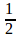,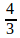,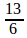, .......

a) What is the common difference?

b) Which is the first integer term in the sequence?

Get Free Study Materials + 1 Week Free Trial of BrainsPrep Class 10 English Medium Tuition

Qn 11.

The algebraic expression of an arithmetic sequence is 6n+1

a) Write the sequence?

b) What is the remainder when the terms are divided by 6?

c) Write the algebraic expression of the sequence obtained by the natural numbers which leaves a remainder 2 on division by 6?

a) 7,13,19,..

b) 1

c) 6n-4

Get Free Study Materials + 1 Week Free Trial of BrainsPrep Class 10 English Medium Tuition

Qn 12.

The sum of first 15 terms of an arithmetic sequence is 300.

a) What is its 8th term?

b)If the first term is 6 find its common difference?

c) Write the algebraic expression of this sequence?

a) 20

b) 2

c) 2n+4

Get Free Study Materials + 1 Week Free Trial of BrainsPrep Class 10 English Medium Tuition

Qn 13.

The algebraic expression of an arithmetic sequence is 5n-3.

a) What is its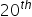term?

b)Find the sum of first 39 terms ?

Get Free Study Materials + 1 Week Free Trial of BrainsPrep Class 10 English Medium Tuition

Qn 14.

The sum of first 5 terms of an arithmetic sequence is 60. Write the sequence?

Mid term =12

For writing the sequence

Get Free Study Materials + 1 Week Free Trial of BrainsPrep Class 10 English Medium Tuition

Qn 15.

a) Write the arithmetic sequence with first term 1 and common difference ½?

b)Find its 31st term?

c) Calculate the sum of its first 31 terms?

Get Free Study Materials + 1 Week Free Trial of BrainsPrep Class 10 English Medium Tuition

Qn 16.

The 10th term of an Arithmetic sequence is 20 and 20th term is 10

a) What is its common difference?

b) Find its 30th term?

a) 10 common difference= - 10

Get Free Study Materials + 1 Week Free Trial of BrainsPrep Class 10 English Medium Tuition

Qn 17.

a. What is the common difference of the arithmetic sequence 20,16,12,..?

b. How many positive numbers are there in this sequence?

c.Which is the first negative number in this sequence?

d. In which position did the first negative number occur in this sequence?

a.common  difference = -4

b.Number of positive terms =  5

c.First negative number

Get Free Study Materials + 1 Week Free Trial of BrainsPrep Class 10 English Medium Tuition

Qn 18.

a)What is the common difference of the arithmetic sequence  -100,-96,-92,...?

b)Check whether Zero is a term in this sequence.

c) Which is the first positive number in this sequence?

a) Common difference = 4

b) Zero is a term.

c) First positive term = 4

Get Free Study Materials + 1 Week Free Trial of BrainsPrep Class 10 English Medium Tuition

Qn 19.

a) Write the arithmetic  sequence with first term 10 and common difference 4?

b)Write the difference of the terms in the same position of the above sequence and the arithmetic  sequence 11,17,23,....?

c) Find the sum of first 20 terms of the sequence thus obtained?

a) 10,14,18,....

b)1,3,5,7,......

c)20²=

Get Free Study Materials + 1 Week Free Trial of BrainsPrep Class 10 English Medium Tuition

Qn 20.

a) Write the sequence of perfect squares which are even.

b) Is it an arithmetic sequence?

a)4,16,36,64,...

b)This is not an arithmetic

Get Free Study Materials + 1 Week Free Trial of BrainsPrep Class 10 English Medium Tuition

Qn 21.

The sum of first 9 terms of an arithmetic sequence is 360.

a)What is its 5th term?

b) If the sum of first 5 terms is 100 ,What is its third term?

c)What is the common difference of this sequence?

d)Write the sequence .

Get Free Study Materials + 1 Week Free Trial of BrainsPrep Class 10 English Medium Tuition

Qn 22.

The sum of first 30 natural numbers is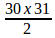.

a) Find the sum of first 30 multiples of 6?

b)Write the algebraic form of the sequence obtained by subtracting 2 from the multiples of 6?

c)Find the sum of first 30 terms of this sequence?

Get Free Study Materials + 1 Week Free Trial of BrainsPrep Class 10 English Medium Tuition

Qn 23.

The algebraic expression of an arithmetic sequence is 8n+5.

a)What is the common difference of this sequence?

b) What is the difference between the smallest four digit number and largest three digit number in this sequence

a) 8

b)

Get Free Study Materials + 1 Week Free Trial of BrainsPrep Class 10 English Medium Tuition

Qn 24.

Consider the arithmetic sequence 4,6,8 ...

a) What is the 5th term in the sequence?

b)Write the ratio between the first and third terms of this sequence?

c)Write the ratio between the second and fifth terms of this sequence?

d)Which term satisfies the same ratio with the 10th term?

a) 12

b) 1:2

c) 1:2

d) 21st term

Get Free Study Materials + 1 Week Free Trial of BrainsPrep Class 10 English Medium Tuition

Qn 25.

a) Which is the first number above 200 which leaves a remainder 3 on division by 7?

b)How many such numbers are there between 200 and 400?

c) Find the sum of these numbers?

a) For finding first number=  206

For finding last number =  395

b) For

Get Free Study Materials + 1 Week Free Trial of BrainsPrep Class 10 English Medium Tuition

Qn 26.

a) Write the first three digit number which is a multiple of 9.

b) Write the sequence of all three digit numbers which are multiples of 9.

c) How many numbers are there in the above sequence ?

d) Find the sum of all these numbers.

Get Free Study Materials + 1 Week Free Trial of BrainsPrep Class 10 English Medium Tuition

Qn 27.

4

8    12

16   20    24

28   32    36   40

... ... ... ... ...

... ... ... ... ... ...

a) Write the next two rows in this pattern .

b) Write the first and last terms of the 11 th row.

Get Free Study Materials + 1 Week Free Trial of BrainsPrep Class 10 English Medium Tuition

Qn 28.

2

4  6   8

10   12   14  16  18

.  .  .  .  .  .  .  .

a)Write the next line in this pattern.

b)How many numbers are there in the 10th row?

c)What are the last and first terms in the tenth row ?

a) 20,22,24,26,28,30, 32

b)20-1=19

c) Identifies the last terms as  2x1², 2x2², 2x3²,...

Get Free Study Materials + 1 Week Free Trial of BrainsPrep Class 10 English Medium Tuition